List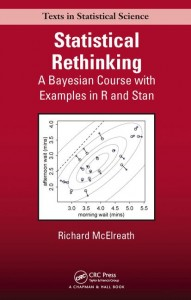Here I work through the practice questions in Chapter 3, “Sampling the Imaginary,” of Statistical Rethinking (McElreath, 2016). I do my best to use only approaches and functions discussed so far in the book, as well as to name objects consistently with how the book does. If you find any typos or mistakes in my answers, or if you have any relevant questions, please feel free to add a comment below.

Here is the chapter summary from page 68:

This chapter introduced the basic procedures for manipulating posterior distributions. Our fundamental tool is samples of parameter values drawn from the posterior distribution. Working with samples transforms a problem of integral calculus into a problem of data summary. These samples can be used to produce intervals, point estimates, posterior predictive checks, as well as other kinds of simulations.

Posterior predictive checks combine uncertainty about parameters, a described by the posterior distribution, with uncertainty about outcomes, as described by the assumed likelihood function. These checks are useful for verifying that your software worked correctly. They are also useful for prospecting for ways in which your models are inadequate.

Once models become more complex, posterior predictive simulations will be used for a broader range of applications. Even understanding a model often requires simulating implied observations. We’ll keep working with samples from the posterior, to make these tasks as easy and customizable as possible.

# Setup
library(rethinking)
set.seed(3)


## Easy Difficulty

These problems use the samples from the posterior distribution for the globe tossing example. This code will give you a specific set of samples, so that you can check your answers exactly. Use the values in samples to answer the questions that follow.

p_grid <- seq(from = 0, to = 1, length.out = 1e3)
prior <- rep(1, 1e3)
likelihood <- dbinom(6, size = 9, prob = p_grid)
posterior <- likelihood * prior
posterior <- posterior / sum(posterior)
samples <- sample(p_grid, prob = posterior, size = 1e4, replace = TRUE)
plot(x = p_grid, y = posterior, type = "l")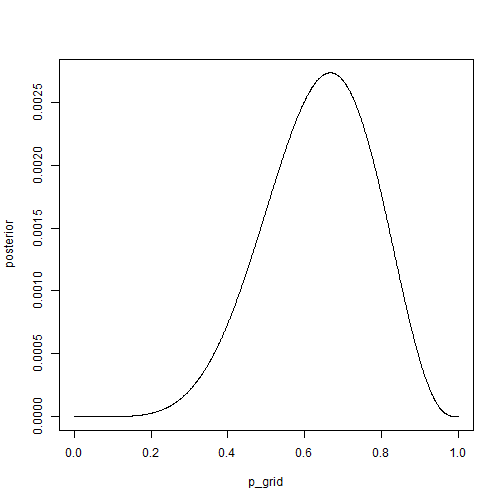### Practice Question 3E1

How much posterior probability lies below $$p=0.2$$?

We need to know what proportion of the values in samples are less than 0.2. The code on page 54 suggests that we use logical indexing, sum these logicals, and divide by the total number of values.

sum(samples < 0.2) / 1e4

##  0.001


However, it would be a bit less code to take the mean of the logicals; this would also reduce the chances of a mistake if the number of samples were to change in the code above. Note that mean() works here in much the same way as sum() does above, treating TRUE values as 1 and FALSE values as 0.

mean(samples < 0.2)

##  0.001


Using the random seed set above, 0.1% of the posterior probability lies below $$p = 0.2$$.

### Practice Question 3E2

How much posterior probability lies above $$p=0.8$$?

The same approach can be used here, simply changing less than 0.2 to greater than 0.8.

mean(samples > 0.8)

##  0.1213


Using the random seed set above, around 12% of the posterior probability lies above $$p = 0.8$$.

### Practice Question 3E3

How much posterior probability lies between $$p=0.2$$ and $$p=0.8$$?

We can use the Boolean & operator to index samples that are greater than 0.2 AND less than 0.8 (as shown on page 54).

mean(samples > 0.2 & samples < 0.8)

##  0.8777


Using the random seed set above, around 88% of the posterior probability lies within [0.2, 0.8].

Note that you could get a very similar answer by subtracting the probabilities calculated in questions 3E1 and 3E2 from 1.

1 - mean(samples < 0.2) - mean(samples > 0.8)

##  0.8777


### Practice Question 3E4

20% of the posterior probability lies below which value of $$p$$?

The quantile() function will allow us to easily find this answer (page 55).

quantile(samples, 0.20)

##       20%
## 0.5193193


20% of the posterior probability lies below $$p=0.52$$.

### Practice Question 3E5

20% of the posterior probability lies above which value of $$p$$?

We can still use the quantile() function but, to get the area above a value, we need to provide its complement.

quantile(samples, 1 - 0.20)

##       80%
## 0.7617618


20% of the posterior probability lies above $$p=0.76$$.

### Practice Question 3E6

Which values of $$p$$ contain the narrowest interval equal to 66% of the posterior probability?

To get the narrowest interval, we need to find the highest posterior density interval (page 56).

HPDI(samples, prob = 0.66)

##     |0.66     0.66|
## 0.5205205 0.7907908


The narrowest interval equal to 66% of the posterior probability is within [0.52, 0.79].

### Practice Questions 3E7

Which values of $$p$$ contain 66% of the posterior probability, assuming equal posterior probability both below and above the interval?

To get the interval that has equal probability mass in each tail, we need to find the percentile interval (page 56).

PI(samples, prob = 0.66)

##       17%       83%
## 0.5005005 0.7747748


The interval of [0.50, 0.77] contains 66% of the posterior probability with equal probability mass both below and above.

## Medium Difficulty

### Practice Question 3M1

Suppose the globe tossing data had turned out to be 8 water in 15 tosses. Construct the posterior distribution, using grid approximation. Use the same flat prior as before.

The grid approximation approach is shown on page 56. We will use a flat prior as instructed and will enter the observed data (8 in 15 tosses) into the dbinom() function as arguments.

p_grid <- seq(from = 0, to = 1, length.out = 1e3)
prior <- rep(1, 1e3)
likelihood <- dbinom(8, size = 15, prob = p_grid)
posterior <- likelihood * prior
posterior <- posterior / sum(posterior)
plot(x = p_grid, y = posterior, type = "l")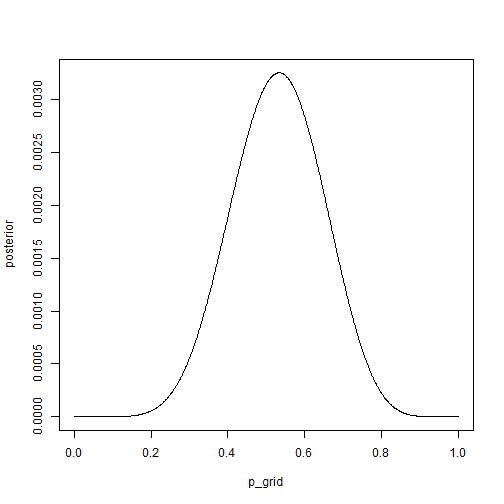### Practice Question 3M2

Draw 10,000 samples from the grid approximation from above. Then use the samples to calculate the 90% HPDI for $$p$$.

We can use the $$sample()$$ function to draw with replacement from the grid approximation.

samples <- sample(p_grid, prob = posterior, size = 1e4, replace = TRUE)
HPDI(samples, prob = .90)

##      |0.9      0.9|
## 0.3293293 0.7147147


The 90% HPDI is thus within [0.33, 0.71].

### Practice Question 3M3

Construct a posterior predictive check for this model and data. This means simulate the distribution of samples, averaging over the posterior uncertainty in $$p$$. What is the probability of observing 8 water in 15 tosses?

To simulate the distribution of samples, we need to use the rbinom() function and provide the appropriate probability distribution (as on page 66).

w <- rbinom(1e4, size = 15, prob = samples)
simplehist(w)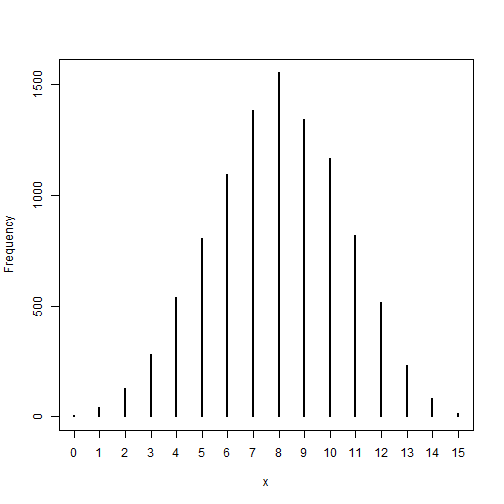mean(w == 8)

##  0.1553


The probability of observing 8 water in 15 tosses is equal to 15.5%.

Judging from the histogram, this is the most likely outcome.

### Practice Question 3M4

Using the posterior distribution constructed from the new (8/15) data, now calculate the probability of observing 6 water in 9 tosses.

We can use the same approach as before, simply updating the number of tosses and observed waters.

w <- rbinom(1e4, size = 9, prob = samples)
simplehist(w)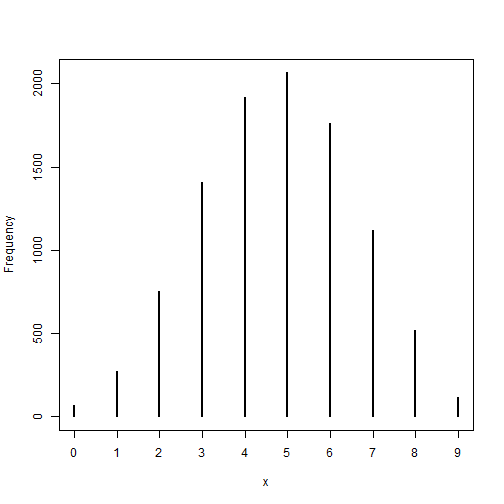mean(w == 6)

##  0.1763


In this case, the probability of observing of 6 water in 9 tosses is 17.6%.

This value is above the modal value (5), but due to there being fewer tosses to spread out the range of possible results, more of the probability mass is here than before.

### Practice Question 3M5

Start over at 3M1, but now use a prior that is zero below $$p=0.5$$ and a constant above $$p=0.5$$. This corresponds to prior information that a majority of the Earth’s surface is water. Repeat each problem above and compare the inferences. What difference does the better prior make? If it helps, compare inferences (using both priors) to the true value $$p=0.7$$.

We’ll use the same approach as before, but replacing the uniform prior with one created by ifelse().

# Redo 1
p_grid <- seq(from = 0, to = 1, length.out = 1e3)
prior <- ifelse(p_grid < .5, 0, 1)
likelihood <- dbinom(8, size = 15, prob = p_grid)
posterior <- likelihood * prior
posterior <- posterior / sum(posterior)
plot(x = p_grid, y = posterior, type = "l")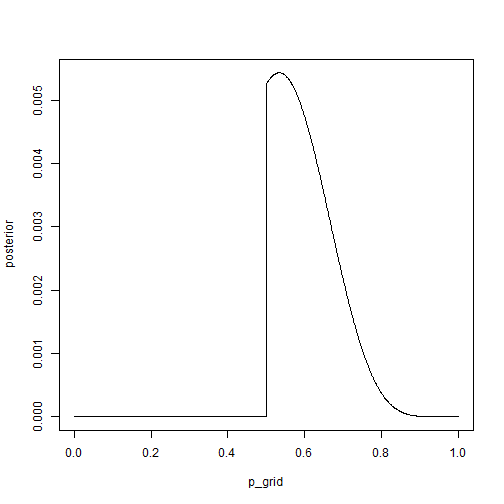This plot looks similar to before (from 3M1) with two notable exceptions: (1) all posterior probability values have become zero for values of $$p<0.5$$, and (2) the height of the remainder of the curve ($$p>0.5$$) has increased (as evidenced by the scaling of the y-axis increasing). Thus, the probability mass that had been assigned to be below $$p=0.5$$ appears to have been shifted up to the remainder of the curve.

# Redo 2
samples <- sample(p_grid, prob = posterior, size = 1e4, replace = TRUE)
HPDI(samples, prob = .90)

##      |0.9      0.9|
## 0.5005005 0.7117117


The prior has a big impact on the lower bound of the HPDI (i.e., increasing it from 0.33 to 0.5). Interestingly, it does not influence the upper bound very much. So the probability mass that was below $$p=0.5$$ must have shifted to be between $$p=0.5$$ and $$p=0.71$$ or else the upper bound would have changed.

# Redo 3
w <- rbinom(1e4, size = 15, prob = samples)
simplehist(w)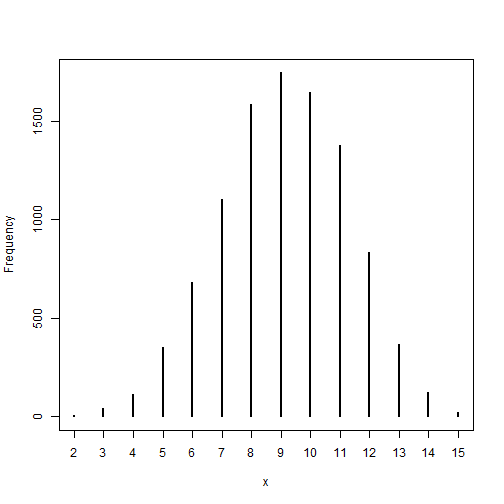mean(w == 8)

##  0.1583


The histogram is now less symmetrical (being lower on the left-hand side), corresponding to a lower probability of observing few waters. The modal predicted value has also shifted from 8 to 9. Combining these effects, the probability of observing 8 water in 15 tosses has increased slightly from 15.5% to 15.8%.

# Redo 4
w <- rbinom(1e4, size = 9, prob = samples)
simplehist(w)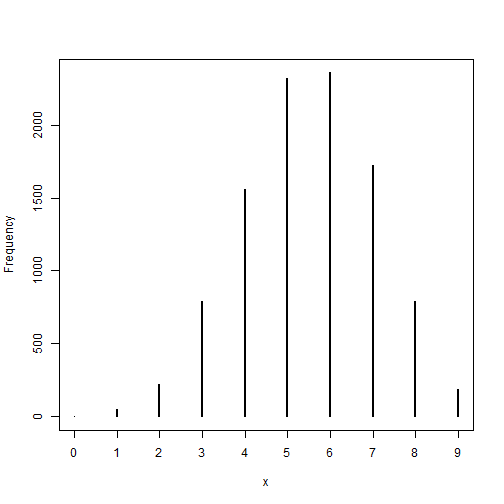mean(w == 6)

##  0.2359


The modal value has shifted from 5 to 6, and the probability of observing 6 water in 9 tosses has increased from 17.6% to 23.6%.

All told, the better prior increased the posterior probability of the true parameter value $$p=0.7$$.

## Hard Difficulty

The practice problems here all use the data below. These data indicate the gender (male=1, female=0) of officially reported first and second born children in 100 two-child families.

data(homeworkch3)
birth1

##    1 0 0 0 1 1 0 1 0 1 0 0 1 1 0 1 1 0 0 0 1 0 0 0 1 0 0 0 0 1 1 1 0 1 0
##   1 1 1 0 1 0 1 1 0 1 0 0 1 1 0 1 0 0 0 0 0 0 0 1 1 0 1 0 0 1 0 0 0 1 0
##   0 1 1 1 1 0 1 0 1 1 1 1 1 0 0 1 0 1 1 0 1 0 1 1 1 0 1 1 1 1

birth2

##    0 1 0 1 0 1 1 1 0 0 1 1 1 1 1 0 0 1 1 1 0 0 1 1 1 0 1 1 1 0 1 1 1 0 1
##   0 0 1 1 1 1 0 0 1 0 1 1 1 1 1 1 1 1 1 1 1 1 1 1 1 1 0 1 1 0 1 1 0 1 1
##   1 0 0 0 0 0 0 1 0 0 0 1 1 0 0 1 0 0 1 1 0 0 0 1 1 1 0 0 0 0


### Practice Question 3H1

Using grid approximation, compute the posterior distribution for the probability of a birth being a boy. Assume a uniform prior probability. Which parameter value maximizes the posterior probability?

We can use the approach detailed on page 56 to calculate the posterior probability and then use which.max() to find the maximum a posteriori estimate (page 59). Because the question is asking about the probability of any birth being a boy, we can combine the information from both birth1 and birth2.

allbirths <- c(birth1, birth2)
p_grid <- seq(from = 0, to = 1, length.out = 1e3)
prior <- rep(1, 1e3)
likelihood <- dbinom(sum(allbirths), size = length(allbirths), prob = p_grid)
posterior <- likelihood * prior
posterior <- posterior / sum(posterior)
plot(x = p_grid, y = posterior, type = "l")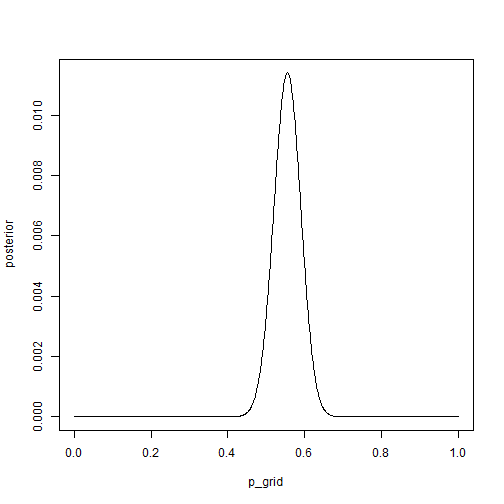p_grid[which.max(posterior)]

##  0.5545546


The parameter value that maximizes the posterior probability is $$p=0.55$$.

### Practice Question 3H2

Using the sample() function, draw 10000 random parameter values from the posterior distribution you calculated above. Use these samples to estimate the 50%, 89% and 97% highest posterior density intervals.

We need to sample with replacement from p_grid using the probability distribution described in posterior. Then we can calculate the requested intervals.

samples <- sample(p_grid, prob = posterior, size = 1e4, replace = TRUE)
HPDI(samples, c(.50, .89, .97))

##     |0.97     |0.89      |0.5      0.5|     0.89|     0.97|
## 0.4794795 0.4964965 0.5265265 0.5725726 0.6066066 0.6256256


The 50% HPDI is $$[0.53, 0.57]$$, the 89% HPDI is $$[0.50, 0.61]$$, and the 97% HPDI is $$[0.48, 0.63]$$.

### Practice Question 3H3

Use rbinom() to simulate 10000 replicates of 200 births. You should end up with 10000 numbers, each one a count of boys out of 200 births. Compare the distribution of predicted numbers of boys to the actual count in the data (111 boys out of 200 births). There are many good ways to visualize the simulations, but the dens() command (part of the rethinking package) is probably the easiest way in this case. Does it look like the model fits the data well? That is, does the distribution of predictions include the actual observation as a central, likely outcome?

We can use the approach detailed on page 66 to conduct this simulation. Then, as suggested by the question, we can visualize the result using dens(). To see where the actual result fits into the posterior predictive distribution, we can add its value using abline().

ppc <- rbinom(1e4, size = 200, prob = samples)
dens(ppc)
abline(v = sum(allbirths), col = "blue")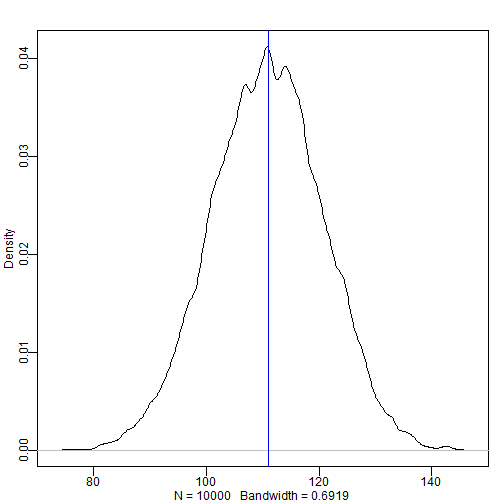The model seems to fit the data well, as the observed count (i.e., 111) is basically the modal value in this distribution.

### Practice Question 3H4

Now compare 10000 counts of boys from 100 simulated first borns only to the number of boys in the first births, birth1. How does the model look in this light?

We need to update the likelihood to consider only first births, recalculate the posterior distribution, and then redo the simulation for 100 births. We can visualize the results and comparison the same way as before.

likelihood <- dbinom(sum(birth1), size = length(birth1), prob = p_grid)
posterior <- likelihood * prior
posterior <- posterior / sum(posterior)
samples <- sample(p_grid, prob = posterior, size = 1e4, replace = TRUE)
ppc <- rbinom(1e4, size = 100, prob = samples)
dens(ppc)
abline(v = sum(birth1), col = "blue")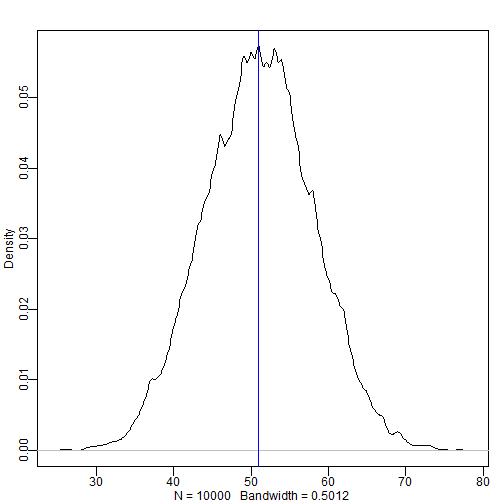The observed value of 51 boys in 100 first borns is consistent with the model, as it is near the center of the probability mass.

### Practice Question 3H5

The model assumes that sex of first and second births are independent. To check this assumption, focus now on second births that followed female first borns. Compare 10000 simulated counts of boys to only those second births that followed girls. To do this correctly, you need to count the number of first borns who were girls and simulate that many births, 10000 times. Compare the counts of boys in your simulations to the actual observed count of boys following girls. How does the model look in this light? Any guesses what is going on in these data?

As the question suggests, we first need to count the number of first borns who were girls and simulate that many births, 10000 times. We can do this using rbinom() as before, using the count as the simulation size.

nfirstgirls <- sum(birth1 == 0)
pcc <- rbinom(1e4, size = nfirstgirls, prob = samples)


Now we can compare the observed number of boy births following girl births through the same visualization techniques used previously.

dens(pcc)
ngirlthenboy <- sum(birth2[birth1 == 0])
abline(v = ngirlthenboy, col = "blue")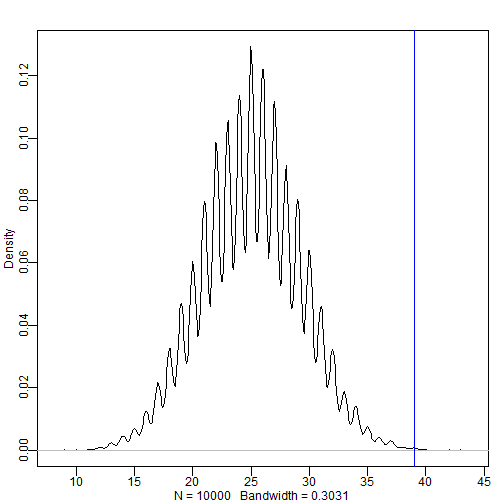The fact that the observed value is so far above the majority of the probability mass suggests that this model is a poor fit to the data. This suggests that sex of first and second birth are not independent. If the data are to be trusted, then this may suggest some biological process that makes male births more likely to follow female births. Alternatively, if the data are biased in some way (e.g., only representing children who live beyond a certain age), then this may suggest some sociocultural or ethological process (e.g., infanticide) that makes females born after females less likely to be included in the data.

### Session Info

sessionInfo()

## R version 3.5.1 (2018-07-02)
## Platform: x86_64-w64-mingw32/x64 (64-bit)
## Running under: Windows >= 8 x64 (build 9200)
##
## Matrix products: default
##
## locale:
##  LC_COLLATE=English_United States.1252
##  LC_CTYPE=English_United States.1252
##  LC_MONETARY=English_United States.1252
##  LC_NUMERIC=C
##  LC_TIME=English_United States.1252
##
## attached base packages:
##  parallel  stats     graphics  grDevices utils     datasets  methods
##  base
##
## other attached packages:
##  knitr_1.21           RWordPress_0.2-3     rethinking_1.59
##  usethis_1.4.0
##
## loaded via a namespace (and not attached):
##   tidyselect_0.2.5   xfun_0.4           purrr_0.2.5
##   lattice_0.20-35    colorspace_1.3-2   htmltools_0.3.6
##   stats4_3.5.1       loo_2.0.0          yaml_2.2.0
##  base64enc_0.1-3    XML_3.98-1.16      rlang_0.3.0.1
##  pkgbuild_1.0.2     pillar_1.3.1       glue_1.3.0
##  withr_2.1.2        bindrcpp_0.2.2     matrixStats_0.54.0
##  bindr_0.1.1        plyr_1.8.4         stringr_1.3.1
##  munsell_0.5.0      gtable_0.2.0       mvtnorm_1.0-8
##  coda_0.19-2        evaluate_0.12      inline_0.3.15
##  callr_3.1.1        ps_1.3.0           markdown_0.9
##  highr_0.7          XMLRPC_0.3-1       Rcpp_1.0.0
##  scales_1.0.0       jsonlite_1.6       mime_0.6
##  fs_1.2.6           gridExtra_2.3      digest_0.6.18
##  stringi_1.2.4      processx_3.2.1     dplyr_0.7.8
##  grid_3.5.1         bitops_1.0-6       cli_1.0.1
##  tools_3.5.1        magrittr_1.5       RCurl_1.95-4.11
##  lazyeval_0.2.1     tibble_1.4.2       crayon_1.3.4
##  pkgconfig_2.0.2    MASS_7.3-50        prettyunits_1.0.2
##  assertthat_0.2.0   rmarkdown_1.11     rstudioapi_0.8
##  R6_2.3.0           compiler_3.5.1


### References

McElreath, R. (2016). Statistical rethinking: A Bayesian course with examples in R and Stan. New York, NY: CRC Press.

### 4 Responses to “Statistical Rethinking: Chapter 3 Practice”

1.rico

Should your prior in M5 not have a probability mass that sums to 1?

prior <- ifelse(p_grid 0 .5

prior_pdf <- ifelse(p_grid < .5, 0, 2)

2.Ryan

Great solutions! Been so helpful having these while I go through the questions in the book

3.Eyas Taifour

Thanks for sharing, very good stuff. it’s good to work on the book exercises and find validation (and explanation) from you, thanks

4.Dan gustafsson

Thanks for sharing! Its great to read someones solutions and compare to my own!